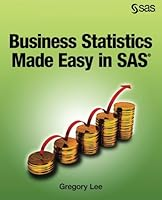0 Reviews
2015-10-28
384 pages

Book Description

"Learn or refresh core statistical methods for business with SAS® and approach real business issues and techniques using a practical approach that avoids complex mathematics and instead employs easy-to-follow explanations. Business Statistics Made Easy in SAS® is designed as a user-friendly, practice-oriented, introductory text to teach businesspeople, students, and others core statistical concepts and applications. It begins with absolute core and takes you through an overview of statistics, data and data collection, an introduction to SAS®, and basic statistics (descriptive statistics and basic associational statistics). The book also provides an overview of statistical , effect size, statistical significance and power , basics of linear regression, introduction to comparison of means, basics of chi-square tests for categories, extrapolating statistics to business outcomes, and some topical issues in statistics, such as big data, simulation, machine learning, and data warehousing. The book steers away from complex mathematical-based explanations, and it also avoids basing explanations on the traditional build-up of distributions, probability theory and the like, which tend to lose the practice-oriented reader. Instead, it teaches the core ideas of statistics through methods such as careful, intuitive written explanations, easy-to-follow diagrams, step-by-step technique implementation, and interesting metaphors. With no previous SAS experience necessary, Business Statistics Made Easy in SAS® is an ideal introduction for beginners. It is suitable for introductory undergraduate classes, postgraduate courses such as MBA refresher classes, and for the business practitioner. It is compatible with SAS® University Edition."

Chapter 1. Introduction to the Central Textbook Example
Chapter 2. Introduction to the Statistics Process
Chapter 3. Introduction to Data
Chapter 4. Data Collection & Capture
Chapter 5. Introduction to SAS®
Chapter 6. Basics of SAS Programs, Data Manipulation, & Reporting
Chapter 7. Descriptive Statistics: Understand your Data
Chapter 8. Basics of Associating Variables
Chapter 9. Using Basic Statistics to Check & Fix Data
Chapter 10. Introduction to Graphing in SAS
Chapter 11. The Statistics Process: Fitting to Data
Chapter 12. Key Concepts: Size & Accuracy
Chapter 13. Introduction to Linear Regression
Chapter 14. Categories Explaining a Continuous Variable: Comparing Two Means
Chapter 15. Categorical Data Distributions & Associations
Chapter 17. Business Analysis from Statistics: Introduction
Chapter 18. Miscellaneous Business Statistics Topics
Chapter 19. Bibliography

Book Details

• Author:
• Length: 384 pages
• Edition: 1
• Language: English
• Publisher:
• Publication Date: 2015-10-28
• ISBN-10: 1629598410
• ISBN-13: 9781629598413